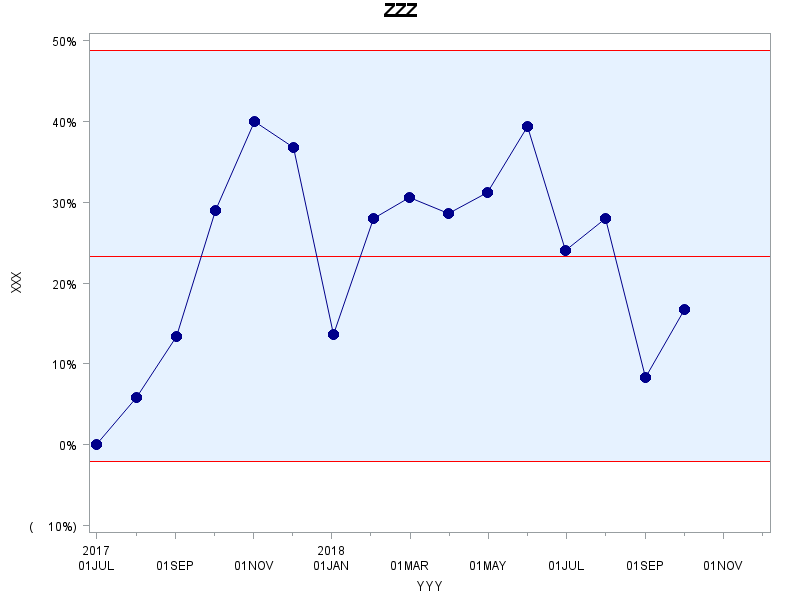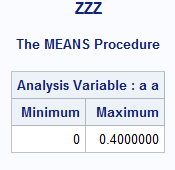## Proc Shewhart - How to set y-axis scale from 0 to 100

I wanted to set the y-axis of a control chart to always run from 0 to 100%. However, Proc Shewhart seems to only allow the data range in y-axis. Is there anyway I can set the y-axis to always run from 0 to 100%?

Here are two options I tried:

``````ods graphics off;
title 'ZZZ';
symbol v=dot color=darkblue h=1.75;
proc shewhart data=percentages;
xchart a*b/ interval = month climits=red nolimitlabel nolegend vformat = percent10. vaxis = (0 to 1 by 0.1);
label a='XXX' b='YYY';
run;

ods graphics off;
title 'ZZZ'; /*TITLE TO THE CHART*/
axis1 order = (0 to 1 by 0.1)
label=("XXX");
axis2 label = ("YYY");
symbol v=dot color=darkblue h=1.75; /*SYMBOL TYPE, COLOR AND DIAMETER*/
proc shewhart data=percentages;
xchart a*b/ vaxis = axis1 haxis=axis2 interval = month climits=red nolimitlabel nolegend vformat=percent10.;
run;``````
1 ACCEPTED SOLUTION

Accepted Solutions

## Re: Proc Shewhart - How to set y-axis scale from 0 to 100

Ok, I think I have discovered the problem.

The lower control limit is negative, so the axis has to have a negative lower limit. However, if your code has

``vaxis=(-0.1 to 1 by 0.1)``

it will work, even if that's not really what you want.

It may be possible to use PCHART which can't have lower limits below 0%, but your data is not set up to allow PCHART to work, you'd probably have to go back to the original data (not the summarized data) to make PCHART work.

--
Paige Miller
11 REPLIES 11

## Re: Proc Shewhart - How to set y-axis scale from 0 to 100

What happened when you tried? Was there an error message or warning in the log? Was a plot actually produced?

What values does the variable A (on the y-axis) contain?

Also, my memory is that you can't have an axis whose range is smaller than the data (unless you CLIP the data), but you should be able to create an axis whose range is larger than the data.

--
Paige Miller

## Re: Proc Shewhart - How to set y-axis scale from 0 to 100

This is what I get when I run the code. The range goes from what seems to be (negative) 10% to 50%, which is the range of the data, although there are no negative values in the data. Variable A (y axis) contains percentages/proportions, and variable B (x axis) contains months. I wanted the y axis to run from 0 to 100.## Re: Proc Shewhart - How to set y-axis scale from 0 to 100

Was there an error message or warning in the log?

--
Paige Miller

## Re: Proc Shewhart - How to set y-axis scale from 0 to 100

``````WARNING: For process variable a the VAXIS= values specified do not cover the data range.
WARNING: Default scaling is substituted``````

## Re: Proc Shewhart - How to set y-axis scale from 0 to 100

That should make you concerned. Please run the following code and provide the calculated minimum and maximum values of A.

``````proc means data=percentages min max;
var a;
format a;
run;``````
--
Paige Miller

## Re: Proc Shewhart - How to set y-axis scale from 0 to 100

This seems about right, the data ranges from 0 to 0.4.## Re: Proc Shewhart - How to set y-axis scale from 0 to 100

Very odd.

How large is this data set PERCENTAGES? If it is not too large, can you attach it here? (Don't bother if it is huge...)

--
Paige Miller

## Re: Proc Shewhart - How to set y-axis scale from 0 to 100

It is a small dataset, I have attached it.

## Re: Proc Shewhart - How to set y-axis scale from 0 to 100

Ok, I think I have discovered the problem.

The lower control limit is negative, so the axis has to have a negative lower limit. However, if your code has

``vaxis=(-0.1 to 1 by 0.1)``

it will work, even if that's not really what you want.

It may be possible to use PCHART which can't have lower limits below 0%, but your data is not set up to allow PCHART to work, you'd probably have to go back to the original data (not the summarized data) to make PCHART work.

--
Paige Miller

## Re: Proc Shewhart - How to set y-axis scale from 0 to 100

Thank you! This does help a lot.

## Re: Proc Shewhart - How to set y-axis scale from 0 to 100

Just as an update tick marks and percentages below 0 on the y-axis can  be suppressed by specifying  values for axis and hiding the tick marks:

``````ods graphics off;
title 'ZZZ';
axis1 order = (-.1 to 1 by 0.1) value=(' ' '0%' '10%' '20%' '30%' '40%' '50%' '60%' '70%' '80%' '90%' '100%')
label=("XXX") major=none;
axis2 label = ("YYY");
symbol v=dot color=darkblue h=1.75;
proc shewhart data=percentages2;
xchart a*b/ vaxis = axis1 haxis=axis2 interval = month climits=red nolimitlabel nolegend vformat=percent10.;
run;``````

Discussion stats
• 11 replies
• 1362 views
• 1 like
• 2 in conversation